# Periodic Classification of Elements- Download Science Study Notes Free PDF For REET/UTET ExamIn many teaching exams including  REETUTET DSSSB Exams etc. Science may be an interesting subject with interesting notes and quizzes. In TET Exams, there will be 30 questions i.e. 15 Questions Science content and 15 questions of Science Pedagogy in REETUTET  and other State TET Exams. Science comprising of various branches of studies like chemistry, physical science, and life science.

Get free Study material for REET Exam

## PERIODIC CLASSIFICATION OF ELEMENTS

Introduction
• Matter around us is present in the form of elements, compounds and mixtures.
• Elements are substances containing atoms of only one type. Eg., Na, Mg, Au etc.
• There are 118 elements known to us. All these have different properties.
• To make the study of these elements easy, these elements have been divided into few groups in such a way that elements in the same group have similar properties.

#### How To Score 25+ in Science For CTET Exam

Dobereiner’s Traids:
When elements were arranged in the order of increasing atomic masses, groups of three elements (known as traids), having similar chemical properties are obtained.
The atomic mass of the middle element of the triad was roughly the average of the atomic masses of the other two elements.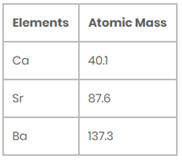Limitations of Dobereiner’s Traids:
Only three traids were recognized from the elements known at that time.
(i) Li, Na, K
(ii) Ca, Sr, Ba
(iii) Cl, Br, I

Newland’s Law of Octaves:
John Newland arranged the known elements in the order of increasing atomic masses and found that the properties of every 8th element are similar to that of the 1st element.
• He compared this to the octaves found in music and called it the “Law of Octaves.” For examples, the properties of lithium (Li) and sodium (Na) were found to be the same.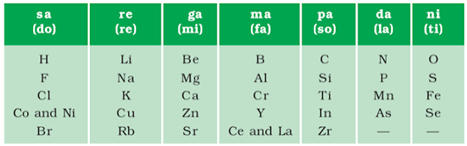Limitations of Newland’s Law of Octaves:
• It was applicable upto Calcium (for lighter elements only).
• Properties of new discovered elements did not fit into the law of octave.

Practice REET Previous Year EVS Quiz For REET Exam Here

Mendeleev’s Periodic Table:
Dmitry Mendeleev, a Russian chemist in 1869 gave Mendeleev’s Periodic Table.
• Till then 63 elements were known.
• He observes the relationship between the atomic masses of the elements and their physical and chemical properties
• It includes 7 periods and 8 groups but inert gases were missing in his table.
• Mendeleev arranged elements in increasing order of their atomic mass.
• He puts elements with similar properties in a group.
• Mendeléev mainly concentrated on the compounds formed by elements with oxygen and hydrogen because they are very reactive and formed compounds in with many elements.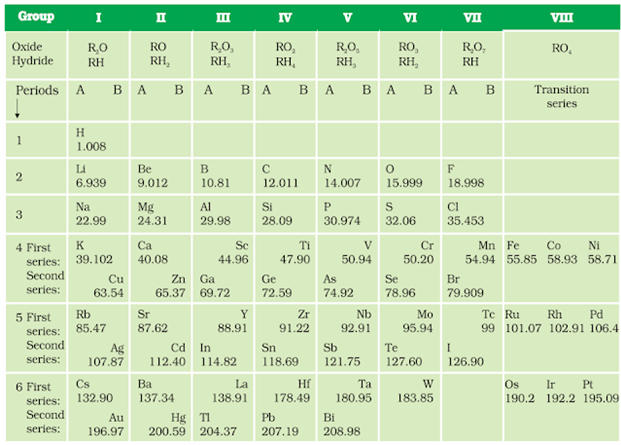Achievements of Mendeleev’s periodic table:
• Gaps left in his periodic table are proved to be useful for the placement of newly discovered elements.
• Later on, some inert gases also placed in this table.
• He also corrected atomic masses of some elements.

REET 2021 : Practice REET Previous Year EVS Quiz For REET Exam Here

Limitations of Mendeleev’s periodic table
• Position of hydrogen is not fixed.
• At some locations, elements were put in order of decreasing atomic mass.
• Position of isotopes could not be explained.

Modern Periodic Table:
• In 1913, Henry Moseley gave the concept of the Modern Periodic Table.
• It states that ‘properties of elements are a periodic function of their atomic number’.
According to the Modern Periodic Law: The properties of elements are a periodic function of their atomic number.
• All the anomalies of Mendeleev’s classification disappear.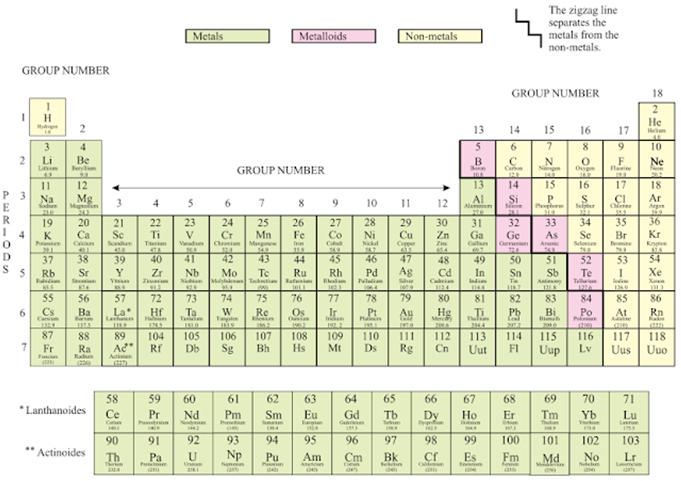Explanation of the Anomalies by Modern Periodic Table:
• Explanation for the position of isotopes (same atomic number put at one place in the same group).
• Cobalt with atomic number 27 came first and nickel (28) should come later.
• Unlike atomic masses, atomic number is always a whole number, so there is no element between hydrogen and helium.
• Atomic number: It is denoted by Z and equal to the number of protons in the nucleus of an atom.
• Modern Periodic table has 18 vertical columns known as ‘groups’ and 7 horizontal rows known as ‘periods’.
• Elements with same number of valance electrons are placed in the same group.
Example: Li: 2,1
Na: 2,8,1
K: 2,8,8,1
• Outermost or valence shell in all three contains 1 electron. These elements have been placed in the same group.
• Number of shells increases as we go down the group.
• Elements with same number of occupied shells are placed in same period.

#### Check Complete Science Study Now: HERE

• For example, Li (2,1); Be(2,2); B(2,3); C(2,4); N(2,5). These elements have same number of shells (two).
• Each period marks a new electronic shell getting filled.
• Number of elements placed in a particular period depends upon the fact that how electrons are filled into various shell.
• Maximum number of electrons that can filled in a shell is given by 2n2 where n is shell number.
• Example:
K shell n = 1 or 2n2 = 2(1)2 = 2 (First period has 2 elements.)
L Shell n = 2 or 2n2 = 2(2)2 = 8 (Second period has 8 elements.)
• Position of an element in the periodic table tells us its chemical reactivity.
• Valence electron determines the kind and number of bonds formed by the elements.

Trends in Modern Periodic Table:

1. Valence e- and valence shell
(a) Across a period:
Valence e- increases from left to right.
Valence shell is constant.
(b) Down the group:
Valence e- remains constant.
Valence shell increases.

2. Valency
(a) Across the period:
Valency increases till group 14 and then decreases till 18.
(b) Down the group:
Valency remains constant.

3. Size of atom
(a) Across the period:
As we move to right, positive charge on nucleus increases, so attraction of outer electron increases. Therefore, electron comes close to nucleus. Thus size of atom decreases from left to right.
(b) Down the group:
As we go down, number of shells increases, so size of atom also increases.

4. Metallic character
(a) Across the period:
Decreases from left to right.
(b) Down the group:
Increases down the group.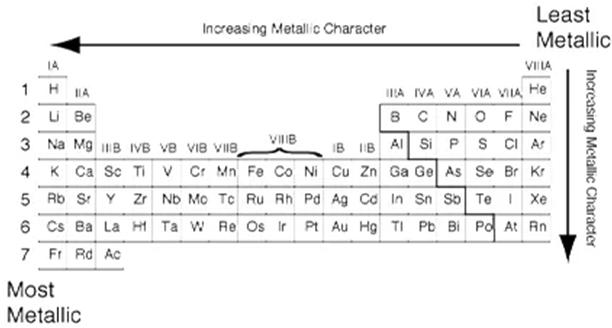5. Electro positivity
• It is the ability of an atom to loose electron.
• If electro positivity is high, it is easy to lose electron.
• If electro positivity is low, it is difficult to loose electron.

#### Check Complete EVS Study Now: HERE

(a) Across the period:
As we move to right, size of atom decreases and therefore more attraction on electrons. So it is difficult to take e- Thus electropositivity decreases from left to right.
(b) Down the group:
As we move down in a group, size of atom increases so less attraction on electrons. So it is easy to take e- Thus electropositivity increases down the group.

6. Nature of oxides
(a) Across the period:
Acidic nature of oxides increases from left to right.
(b) Down the group:
Acidic nature of oxides decreases down the group.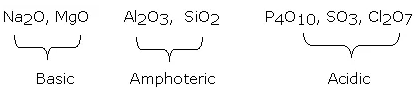7. Chemical reactivity
(a) Across the period:
First decreases as it is more difficult to lose more e- and then increases from left to right as it is easier to gain lesser number of e-.
(b) Down the group:
As we move down in a group, chemical reactivity increases for metals and increases for non-metals.

•Learning Curve - What is Learning Curve?...
•रस - परिभाषा, भे�...
•पठन कौशल- परिभा�...
•अलंकार - परिभाष�...
•Indian States and Capitals 2022: 28 Stat...
•New Education Policy(NEP) PDF - नई �...